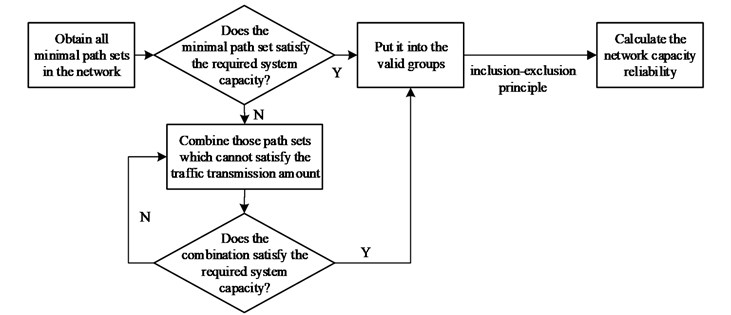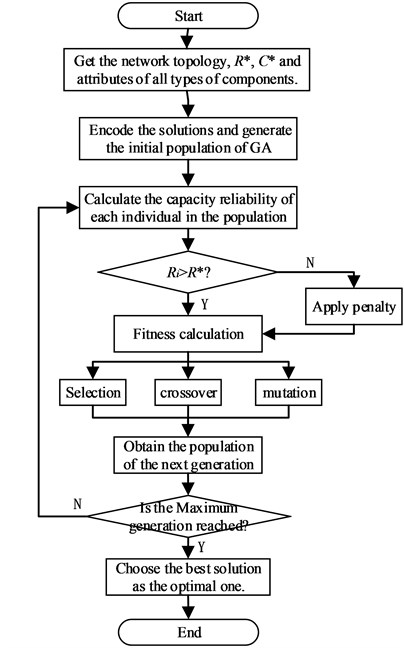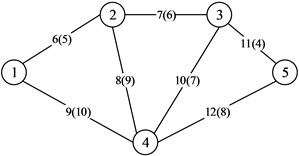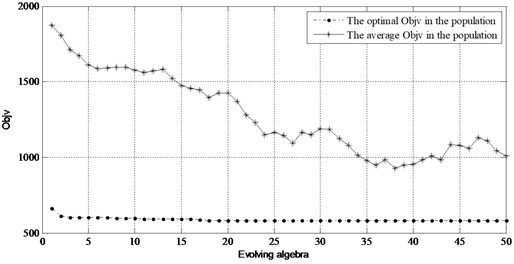Published: 03 November 2014

# Optimal capacity reliability design of networks based on genetic algorithm

Jingfu Wang1
Ruiying Li2
Xiang Li3
Ning Huang4
1, 2, 3, 4School of Reliability and Systems Engineering, Beihang University, Beijing, China
2, 4Science and Technology on Reliability and Environmental Engineering Laboratory, Beijing, China
Corresponding Author:
Ruiying Li
Views 53

#### Abstract

The cost, capacity and reliability of components vary in different component types, and the optimal component combination is determined by minimizing the total cost under the constraint of network capacity reliability requirement. To solve the problem that the gradient method can only be applied for networks whose capacity and reliability of components monotonically increase with the cost, a general optimization model is presented, and a Genetic Algorithm (GA) method using the minimal path sets to calculate the network capacity reliability is proposed to solve this optimal capacity reliability design problem. The optimal types of both nodes and links can be obtained using our optimization method. Our case study on ARPA network shows that our algorithm is efficient for the problem with good convergence and search performance.

## 1. Introduction

Networks are complex nonlinear systems with dynamical failure behavior. For networks with limited capacity, the network reliability is evaluated using capacity reliability . The capacity reliability of networks  is the probability that the network can transmit a given amount of traffic in a given time. In the network design, as reliability, capacity and cost of components vary in different component types, how to select the proper network components, including nodes and links, becomes a problem. Optimal network capacity reliability design becomes a hot research topic. Its goal is to minimize the total cost which can satisfy the network capacity reliability requirement, or maximize the network capacity reliability under the total cost constraint .

Kumar and Ahuja  proposed an optimization method by minimizing both total cost and transmission delay of the communication network under the constraint of capacity reliability requirement. However, the decision variables of this problem are network topology, node locations and link paths, but not component type. Kumar et al.  optimized the throughput between source and destination under the constraint of total cost, and the links capacity and node storage size were determined. However, all link reliability is set as a fixed value in the network, which is not the real situation. With the objective of minimizing the cost, Goyal and Misra  presented an optimal network design problem by constraining on network capacity reliability, and proposed a general gradient method to solve this optimization problem. However, this optimization method can only be applied to component candidates whose reliability and capacity monotonically increase along with the cost. Moreover, the search space of the gradient method is so limited that it may easily fall into local optimal solution.

To solve the above problems, a new optimal network capacity reliability design method is proposed in this paper. Section 2 presents the optimization model, and Section 3 describes the optimization method based on the Genetic Algorithm (GA) method. In Section 4, the ARPA network is used as the case to verify the efficient of our proposed method compared with the general gradient one. Finally, Section 5 concludes our results.

## 2. Optimal capacity reliability design model

For networks with limited capacity, network capacity reliability needs to be considered in the optimal network design. Our goal is to minimize total cost of the network under the constraint of the network capacity reliability requirement. The decision variables are component types, which determines the component reliability, capacity and cost. In this paper, we study the optimal network capacity reliability design based on the following assumptions (which is also frequently used in traditional optimal reliability design problems ):

1) The capacity of both nodes and links is limited in the network;

2) Nodes and links have only two states, fault and normal;

3) The failure probability of nodes and links in the network is statistically independent.

In this problem, the following parameters are already given, including network capacity reliability requirement ${R}^{\mathrm{*}}$, the source and destination of the transmission, the required system capacity ${C}^{\mathrm{*}}$, and the network topology. The optimization model can be presented as:

1
$\begin{array}{l}\mathrm{m}\mathrm{i}\mathrm{n}\mathrm{}\mathrm{}\mathrm{}\mathrm{}g\left({v}_{ij},{l}_{ij},{x}_{ij}\right)\\ s.t.\mathrm{}\mathrm{}\mathrm{}\mathrm{}\mathrm{}\mathrm{}f\left({w}_{ij},{l}_{ij},{x}_{ij}\right)>{R}^{\mathrm{*}}，\\ \mathrm{}\mathrm{}\mathrm{}\mathrm{}\mathrm{}\mathrm{}\mathrm{}\mathrm{}\mathrm{}\mathrm{}\mathrm{}\mathrm{}\mathrm{}\mathrm{}\sum _{j}{x}_{ij}=1，{x}_{ij}\in \left\{0,1\right\}，i\in \left\{1,...,n\right\},\mathrm{}\mathrm{}\mathrm{}j\in \left\{1,2,...\right\},\end{array}$

where $n$ is the number of components (including both links and nodes), $i$ is the component number, $j$ is the type number. For the component $i$ with type $j$, ${v}_{ij}$ is its cost, ${w}_{ij}$ is its reliability, and ${l}_{ij}$ is its length for links, or ${l}_{ij}=$1 for nodes. $g\left({v}_{ij},{l}_{ij},{x}_{ij}\right)$ is the total cost, the sum of all component cost, and it can be calculated as:

2
$g\left({v}_{ij},{l}_{ij},{x}_{ij}\right)=\sum \left({v}_{ij}\cdot {l}_{ij}\cdot {x}_{ij}\right).$

$f\left({w}_{ij},{l}_{ij},{x}_{ij}\right)$ is the calculation of capacity reliability. The capacity reliability calculation method proposed by Aggarwal is applied in this problem. It is an early method but can effectively estimate the reliability of networks with limited capacity . The topology and capacity of networks are modeled via connection matrix. All valid groups (i.e., path sets, including the minimal path sets and their combinations that can satisfy the required system capacity) can be obtained by the algorithm. The capacity reliability is computed based on the valid groups using the principle of inclusion-exclusion  as follows:

3
$P\left(\bigcup _{i=1}^{m}{A}_{i}\right)=\sum _{i=1}^{m}P\left({A}_{i}\right)-\sum _{i,j:i
$\mathrm{}\mathrm{}\mathrm{}\mathrm{}\mathrm{}\mathrm{}+\sum _{i,j,k:i

where $m$ is the number of minimal path sets, event ${A}_{i}$ is the occurrence of minimal path set $i$, and the value range of $i$, $j$, $k$ is [1, $n$]. The detail flow diagram of the method is shown in Fig. 1.

## 3. Optimization based on genetic algorithm

GA provides a generic solution for the system optimization problem. It searches global optimal solutions by the population iteration. It is applicable for large scale and high nonlinear optimization problems without analytical expressions . Different from traditional single-point search algorithm, GA can handle multiple individuals in the search space simultaneously and evaluate multiple solutions simultaneously . So it has better global search capability. These features make GA capable to solve such complex problems as optimal network capacity reliability design.

Fig. 1The calculation method of capacity reliability### 3.1. The procedure of GA

In this paper, GA is used to solve the optimization model in Eq. (1). The procedure of the optimal capacity reliability design based on GA is shown in Fig. 2.

Fig. 2Flowchart of optimal network capacity reliability design based on genetic algorithmFor a network with $n$ components, decision variables of GA are [${S}_{1}$, ${S}_{2}$, …, ${S}_{n}$], where ${S}_{i}$ is the selected type number of the $i$th component. These variables are encoded, and the initial population is randomly generated. The fitness of each individual is calculated, and then use selection, crossover and mutation operations to generate the next generation. Some possible choices of selection, crossover and mutation operations are listed in Table 1.

These procedures are iterated until the maximum number of the generation is achieved. Finally, the component combination with the minimum cost is determined as the optimal solution.

Table 1Operations of selection, crossover and mutation

 Items Set Selection Roulette selection, random universal selection, elitism strategy Crossover Single-point crossover, two-point crossover, multi-point crossover Mutation Simple mutation, uniform mutation, boundary mutation

### 3.2. The fitness function

The fitness function is used to evaluate the fitness of each individual, which is important in generating the next generation. For individuals those can satisfy the capacity reliability requirement, a high fitness value is given, and vice versa. The fitness function can be used to select the applicable individuals and eliminate others .

From Eq. (1), our objective is to achieve the minimum total cost under the constraint of the capacity reliability requirement. For those component combinations which cannot satisfy the capacity reliability requirement, the penalty function  is defined as:

4
$P\left(x\right)=\left\{\begin{array}{ll}0，& R\ge {R}^{\mathrm{*}}，\\ {P}_{f}，& R<{R}^{\mathrm{*}},\mathrm{}\mathrm{}\mathrm{}R\ne 0,\\ {P}_{f}^{\mathrm{\text{'}}}，& R=0，\end{array}\right\$

where ${P}_{f}$ is a value greater than the largest possible cost of an individual, ${P}_{f}^{\mathrm{\text{'}}}>{P}_{f}$, and $R$ is capacity reliability of the individual (i.e., the component combination). If no valid group exist, $R=$ 0.

Based on the penalty function, the objective function used to calculate the fitness can be computed as:

5
$ObjV=g\left({v}_{ij},{l}_{ij},{x}_{ij}\right)-P\left(x\right),$

where $g\left({v}_{ij},{l}_{ij},{x}_{ij}\right)$ is the total cost of the individual.

According to Eqs. (3) and (4), if the capacity reliability of the individual cannot reach the required value, its objective function value is lower than those can satisfy the requirement. If the capacity reliability is 0, the objective function value is even lower. This ensures individuals that cannot satisfy the capacity reliability requirement can be eliminated in the evolution as soon as possible.

The cost of different component combinations may vary greatly. It may not reflect the average performance of the population when $ObjV$ is directly used as the fitness function. To solve the problem, the linear fitness scaling based on sequence  is applied in this paper. All individuals are ordered by $ObjV$ from small to large, and their fitness can be calculated as:

6
$FitnV\left(Pos\right)=2-sp+2×\left(sp-1\right)×\frac{Pos-1}{PopSize-1},$

where $PopSize$ is the population size, $Pos$ is the sequence number of individuals in the population, $sp$ is the selection pressure, of which value range is [1, 2]. The selection pressure determines the size of transformation scale, and its default value is 2. This linear fitness scaling method changes the fitness gap between different individuals, and also keeps the population diversity.

## 4. Case study

The ARPA network shown in Fig. 3 is used as an example to verify the effectiveness of our proposed method. Assume that 50 units of traffic is required to be transmitted from Node 1 to Node 5. Let the capacity reliability requirement of the network be 0.98. Attributes of possible types of components are shown in Table 2.

From Table 2, one can see that the capacity is not always increased with the cost, and the gradient method in  cannot be used. Our method with the following parameters are used to find the optimal solution.

The optimal result can be obtained in Table 4, and the optimization process is shown in Fig. 4. One can see that both the average and optimal values of the objective function in the population decline gradually. It shows that GA has good convergence and search performance in solving this problem.

Fig. 3The topology of the ARPA network: Note: (1) the number in the circle is the node number; (2) the number out of parentheses is the link number; and (3) the number in parentheses is the link lengthFig. 4Optimization process with genetic algorithmTable 2Attributes of possible types of components

 Component type number Category Capacity Cost (per unit length) Reliability (per unit length) 1 Node 45 60 0.9980 2 Node 60 50 0.9960 3 Node 50 40 0.9930 4 Node 40 30 0.9900 5 Link 64 10 0.9998 6 Link 55 9 0.9996 7 Link 46 8 0.9995 8 Link 32 7 0.9993 9 Link 16 5 0.9990

Table 3Parameters of the genetic algorithm

 Population size Number of generations Selection operator Crossover operator Crossover probability Mutation operator Mutation probability 100 50 Roulette selection with elitism strategy Single-point crossover 0.7 Discrete mutation 0.03

The optimization results show that: (1) the GA is applicable for solving optimal network capacity reliability design problems and has good convergence, and (2) compared to the gradient method, the GA can be applied to those component candidates whose capacity and/or reliability do not increase with cost.

Table 4The optimal solution

 Component number Capacity reliability Total cost 2, 3, 3, 3, 3, 2, 3, 5, 2, 5, 2, 2 0.9809 581

## 5. Conclusions

In this paper, an optimization method based on genetic algorithm is proposed for optimal network capacity reliability design problem. The optimization model is presented, and the GA optimization procedure and its fitness function is detailed addressed. Our proposed method can be used for component candidates whose capacity and/or reliability is not monotonically increase with the cost.

It is not easy to calculate the capacity reliability for large scale networks, the related topics will be studied in our future research.